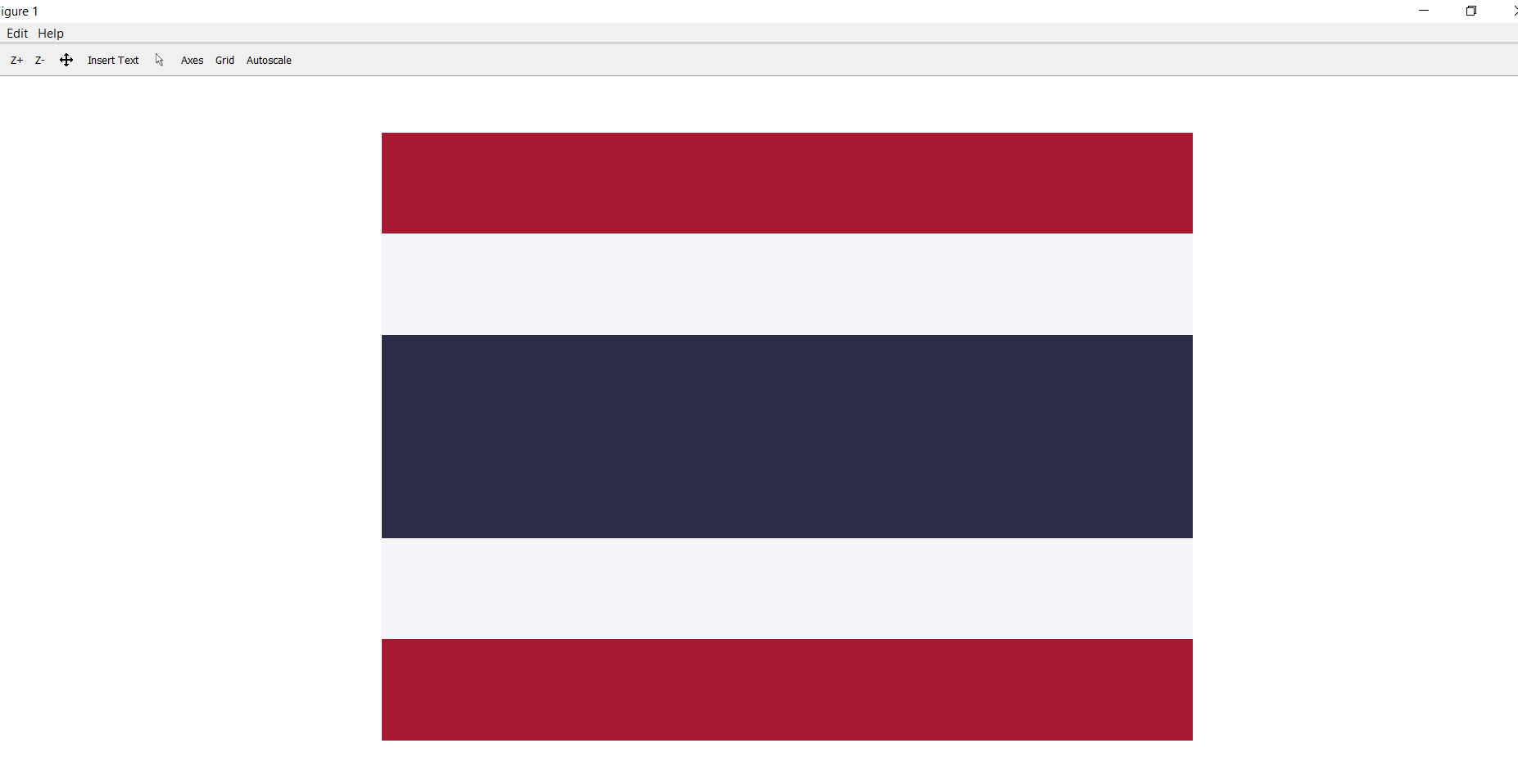Open in App
Not now

# Draw Thailand Flag Using MATLAB

• Last Updated : 10 Apr, 2019

Prerequisite: RGB image representation

A coloured image can be represented as a 3 order matrix. The first order is for the rows, the second order is for the columns and the third order is for specifying the colour of the corresponding pixel. Here we use the RGB colour format, so the third order will take 3 values of Red, Green and Blue respectively. The values of the rows and columns depending on the size of the image.

The flag of Thailand shows five horizontal stripes in the colours red, white, blue, white and red, with the central blue stripe being twice as wide as each of the other four.

Steps:

1. First, we make a matrix of dimensions 600 X 800 X 3. Where the number of pixels of rows is 600, the number of pixels of columns is 800 and 3 is the colour coding in RGB format.
2. Paint the first and the last strip with red. RGB is (165, 25, 49).
3. Paint the middle strip with blue. RGB is (45, 44, 72).
4. Paint the remaining two strips with white. RGB is (244, 245, 248).

Below is the code:

 `I = zeros(600, 800, 3);``% here image is of class ‘uint8’, the range of values ``% that each colour component can have is [0 – 255] ``I= uint8(I);`` ` `%Painting the Red Strip``I(1:100, :, 1)=165;``I(1:100, :, 2)=25;``I(1:100, :, 3)=49;`` ` `%Painting the White Strip``I(101:200, :, 1)=244;``I(101:200, :, 2)=245;``I(101:200, :, 3)=248;`` ` `%Painting the Blue Strip``I(201:400, :, 1)=45;``I(201:400, :, 2)=44;``I(201:400, :, 3)=72;`` ` `%Painting the white Strip``I(401:500, :, 1)=244;``I(401:500, :, 2)=245;``I(401:500, :, 3)=248;`` ` `%Painting the Red Strip``I(501:600, :, 1)=165;``I(501:600, :, 2)=25;``I(501:600, :, 3)=49;`` ` `%Show the image formed``figure;imshow(I);`

Output:My Personal Notes arrow_drop_up